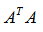# 向量与矩阵的范数（比较1-范数、2-范数、无穷范数、p-范数、L0范数 和 L1范数等）阅读文献时，经常看到各种范数，机器学习中的稀疏模型等，也有各种范数，其名称往往容易混淆，例如：L1范数也常称为“1-范数”，但又和真正的1-范数又有很大区别。下面将依次介绍各种范数。

## 1、向量的范数

向量的1-范数：  ; 各个元素的绝对值之和；

向量的2-范数：；每个元素的平方和再开平方根；

向量的无穷范数：

p-范数：，其中正整数p≥1，并且有

Matlab代码：X=[2, 3, -5, -7]; XLfs1=norm(X,1);

Matlab代码：X=[2, 3, -5, -7]; XLfs2=norm(X,2);

（1）正无穷范数：向量的所有元素的绝对值中最大的；即X的正无穷范数为：7；

Matlab代码：X=[2, 3, -5, -7]; XLfsz=norm(X,inf);

（2）负无穷范数：向量的所有元素的绝对值中最小的；即X的负无穷范数为：2；

Matlab代码：X=[2, 3, -5, -7]; XLfsf=norm(X,-inf);

## 2、矩阵的范数

A=[2, 3, -5, -7;

4, 6,  8, -4;

6, -11, -3, 16];

（1）矩阵的1-范数（列模）：；矩阵的每一列上的元素绝对值先求和，再从中取个最大的，（列和最大）；即矩阵A的1-范数为：27

Matlab代码：fs1=norm(A,1);

（2）矩阵的2-范数（谱模）：，其中   的特征值；矩阵的最大特征值开平方根。

Matlab代码：fs2=norm(A,2);

（3）矩阵的无穷范数（行模）：；矩阵的每一行上的元素绝对值先求和，再从中取个最大的，（行和最大）

Matlab代码：fswq=norm(A,inf);

下面要介绍关于机器学习中稀疏表示等一些地方用到的范数，一般有核范数，L0范数，L1范数（有时很多人也叫1范数，这就让初学者很容易混淆），L21范数（有时也叫2范数），F范数等，这些范数都是为了解决实际问题中的困难而提出的新的范数定义，不同于前面矩阵的范数。

http://www.cnblogs.com/MengYan-LongYou/p/4050862.html

https://blog.csdn.net/u013066730/article/details/51145889

http://blog.sina.com.cn/s/blog_7103b28a0102w73g.html

（4）矩阵的核范数：矩阵的奇异值（将矩阵svd分解）之和，这个范数可以用来低秩表示（因为最小化核范数，相当于最小化矩阵的秩——低秩）；

Matlab代码：JZhfs=sum(svd(A));

（5）矩阵的L0范数：矩阵的非0元素的个数，通常用它来表示稀疏，L0范数越小0元素越多，也就越稀疏。

（6）矩阵的L1范数：矩阵中的每个元素绝对值之和，它是L0范数的最优凸近似，因此它也可以近似表示稀疏；

Matlab代码：JZL1fs=sum(sum(abs(A)));

（7）矩阵的F范数：矩阵的各个元素平方之和再开平方根，它通常也叫做矩阵的L2范数，它的有点在它是一个凸函数，可以求导求解，易于计算；

Matlab代码：JZFfs=norm(A,'fro');

（8）矩阵的L21范数：矩阵先以每一列为单位，求每一列的F范数（也可认为是向量的2范数），然后再将得到的结果求L1范数（也可认为是向量的1范数），很容易看出它是介于L1和L2之间的一种范数

Matlab代码：JZL21fs=norm(A(:,1),2) + norm(A(:,2),2) + norm(A(:,3),2)++ norm(A(:,4),2);

### Matlab代码

clear all;clc;

%% 求向量的范数
X=[2, 3, -5, -7];   %初始化向量X
XLfs1=norm(X,1);    %向量的1-范数
XLfs2=norm(X,2);    %向量的2-范数
XLfsz=norm(X,inf);  %向量的正无穷范数
XLfsf=norm(X,-inf); %向量的负无穷范数

%% 求矩阵的范数
A=[2, 3, -5, -7;
4, 6,  8, -4;
6, -11, -3, 16];     %初始化矩阵A

JZfs1=norm(A,1);        %矩阵的1-范数
JZfs2=norm(A,2);        %矩阵的2-范数
JZfswq=norm(A,inf);     %矩阵的无穷范数
JZhfs=sum(svd(A));      %矩阵的核范数
JZL1fs=sum(sum(abs(A)));% 矩阵的L1范数
JZFfs=norm(A,'fro');    %矩阵的F范数
JZL21fs=norm(A(:,1),2) + norm(A(:,2),2) + norm(A(:,3),2)++ norm(A(:,4),2);% 矩阵的L21范数

### 参考资料

1. 微信公众号:2. CSDN博客：https://xiongyiming.blog.csdn.net/

01-09343707-131万+
03-169973
07-185万+
09-233万+
09-275万+
06-27964
06-2416万+
03-316317
10-25331
©️2020 CSDN 皮肤主题: Age of Ai 设计师:meimeiellie